´

# Introduction

Up to now, there are only finitely many Steiner 5-designs known and, with one exception of a 5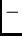(36,6,1) design , they all admit some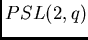as a group of automorphisms. But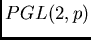is not admitted by a result of Denniston . So, we here analyse the special situation of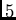-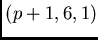designs with automorphism group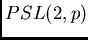for some prime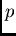. In addition, we only consider such designs that are assembled from orbits with non-trivial stabilizers. This restriction has already been introduced by Denniston  and was used by Grannell, Griggs and Mathon  and Betten, Laue, Molodtsov, Wassermann . We show that these orbits of 6-element subsets can be constructed very fast by computer and that one also can get the information on the orbits of 5-element subsets that are contained in a 6-element subset from a fixed orbit. This is the information usually needed for a general backtrack solver to collect orbits for a design. The approach is an obvious refinement of the widely used methods of Kramer and Mesner  where all orbit incidences are kept in a matrix, called the Kramer-Mesner matrix now.

There are two techniques employed for this approach. Firstly, orbit calculations are replaced by fixed point determination like in Polya's theory of counting. We already made use of this idea in solving isomorphism problems for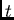-designs with prescribed automorphism group . Secondly, the idea of using cross-ratio's as an invariant for the 5-orbits employed by Alltop  for 4-orbits, see also , is refined from-orbits to-orbits. This variation is worked out for the case of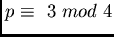, the only case where-designs with automorphism groupmay exist. Here the Legendre symbol has to be evaluated for a certain ratio in addition to the ordinary cross ratio.

There are some divisibility conditions and group theoretic results that reduce the possibilities for-designs with automorphism groupto exist. The number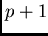of points has to be divisible by 12 and if 5 divides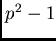then 5 divides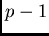. Also, the stabilizer of a block of a-design inmay only have an order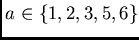. Sinceis 3-homogeneous if, each orbit is a 3-design. If all orbits that form a-design are of the same length we have a partition of the-design into 3-designs all having the same parameters. These might be useful for constructions like the famous Large Set recursions. If we look for designs formed by orbits of the same length then we can use additional restrictions. We already considered the case of orbits of length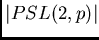in . Then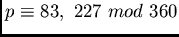. We here show that the only further possibility is that all orbits have length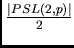and then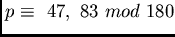. We have found such designs for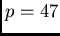and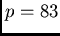. Prescribing a stabilizer of order 2 reduces the problem size drastically, but the next case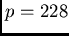still is too large for our presently used solver.

The methods are implemented by an algorithm written in C. We make use of some special shortcuts that only can be applied in a search for Steiner systems. In particular, only those columns of the Kramer-Mesner matrix are kept which have only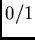-entries and duplicate columns are avoided. The matrix is kept as a sparse matrix and the invariant of a 5-element orbit is used for directly addressing the orbit. No data structure is needed for the big group. So, the computation time is linear in the number of orbits on 5-element sets and 6-element sets constructed. The program allows to prescribe any combination of non-trivial stabilizers. We have collected some statistics in a table. The first author thanks Gene Cooperman for inspiring discussions and a fruitful time at the Northeastern University that initiated this research.Next: Basic Conditions Up: Partitioned Steiner 5-Designs Previous: Partitioned Steiner 5-Designs
N.N. 2002-02-25

University of Bayreuth -